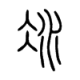用JQuery做一个Ajax星星评分功能

``` class rater(models.Model): counts = models.IntegerField() #记录评分次数 score = models.FloatField() #记录分数 ```

``` def rate_json(request,item_id): #该函数返回给定item的分数,评分次数,总分。通过url:/rate_json/item_id/访问 r = rater.objects.get(item__id=int(item_id)) data = {} data['counts'] = i.counts data['score_sum']= i.score*i.counts data['score'] = r.score return HttpResponse(json.dumps(data), content_type="application/json") #返回一个json格式数据 ```

``` def rate_item(request,item_id,score): #处理用户提交分数。通过url:/rate_json/item_id/score/访问 score = int(score) if (score<=5 and score>=0): i = rater.objects.get(item__id=int(item_id)) score_sum = i.counts * i.counts i.counts += 1 i.score = score_sum / i.counts i.save() return rate_json(request,item_id) ```

3 Replies to “用JQuery做一个Ajax星星评分功能”

1.Louis Hansays:

看起来挺有意思的

2.大Dsays:

等了一万年，终于更新了

1.ericsays:

哈哈 。 。 。 争取经常更新